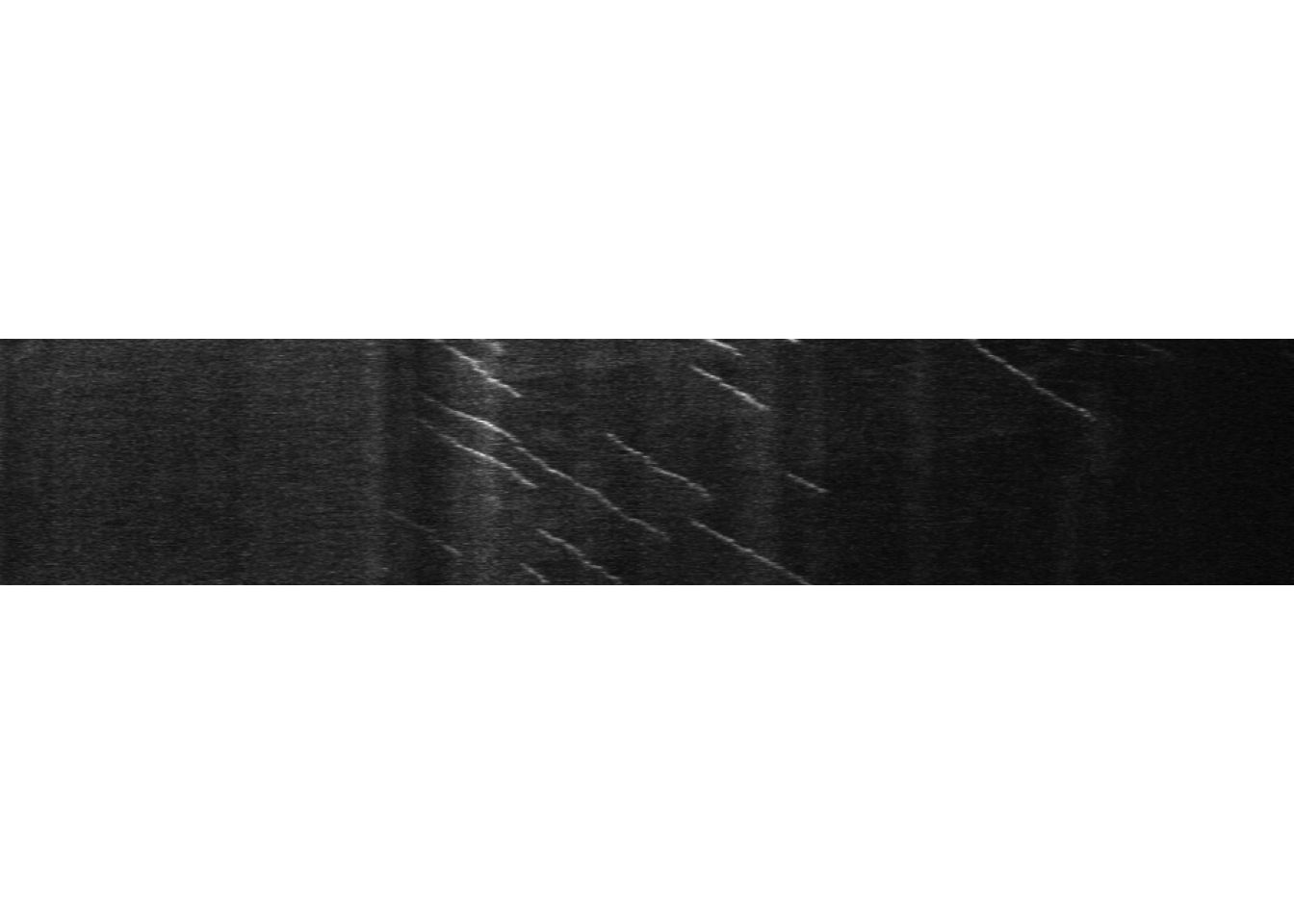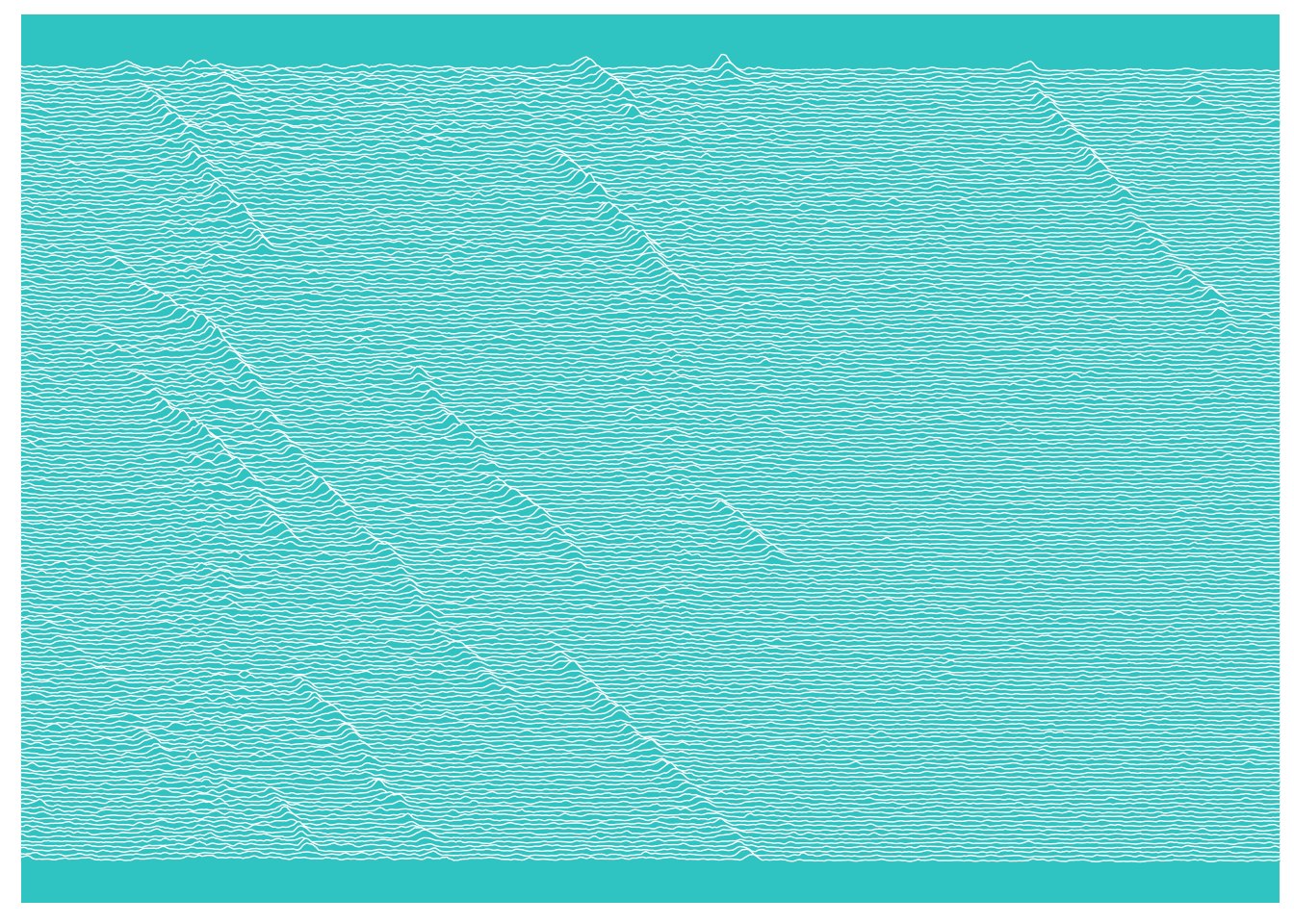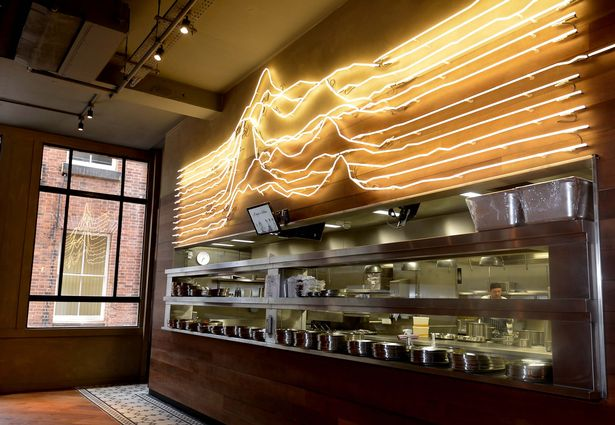I have a bit of a love hate relationship with kymographs. In the way that they compress data there’s no doubt that you loose information, but in the world of axonal transport and low signal:noise they have clear advantages in enabling quantification.

I covered before a couple of strategies you can use to import image data into R. The next step in my workflow is usually to turn that image into a data table for further analysis. A data table can take many forms, but I think the easiest and most powerful way to get the most out of R these days is to use the tidyverse collection of packages.

I picked up R a few years ago now and since then I’ve happily watched the tidyverse grow and develop. It is a fantastic set of tools accessible to anyone, and it’s easy to see the parallels between ImageJ/FIJI development and the R/tidyverse. To work happily within the tidyverse your data does have to be tidy. Tidy data is a standardized way of mapping the meaning of data to it’s structure (across lots of different types of data). A simple concept in essence, I remember it taking me a while to fully transition from spreadsheets until the penny really dropped. Tidy data is data that conforms to the following rules:

1. Each variable describing the data forms a separate column.
2. Each observation forms a row and all the parameters you need to describe that observation should be on that row.
3. Each type of observational unit forms a table.

This is my strategy to turn an image into tidy data for downstream analysis. First up, load the packages we need. The `tidyverse` package loads a suite of (currently) 26 packages, but in this example I’m only actually using functions from `tibble`, `tidyr`, `forcats` and `ggplot2`. I’m also loading `EBImage` to import my data:

``````library(tidyverse)
library(EBImage)

kymograph <- readImage("MAX_Reslice_of_low_pass_axon_16bit.tif", type = "tiff")
display(kymograph, method = "raster")``````This image is 950 pixels wide and 181 pixels high.

Turning the image into a data table is easy enough, in this case I will turn it into a tibble - the tidyverse class for storing a data frame - which is faster than `as.data.frame()` used in base R.

``````#' Turn the kymograph tif into a tibble
kymo_data <- as_tibble(kymograph)``````
``````## Warning: Calling `as_tibble()` on a vector is discouraged, because the behavior is likely to change in the future. Use `tibble::enframe(name = NULL)` instead.
## This warning is displayed once per session.``````
``````#' Take a quick peek
kymo_data``````
``````## # A tibble: 950 x 181
##       V1    V2    V3    V4    V5    V6    V7    V8    V9   V10   V11   V12
##    <dbl> <dbl> <dbl> <dbl> <dbl> <dbl> <dbl> <dbl> <dbl> <dbl> <dbl> <dbl>
##  1 0.228 0.153 0.253 0.195 0.215 0.308 0.251 0.322 0.277 0.258 0.185 0.278
##  2 0.195 0.165 0.238 0.190 0.230 0.284 0.234 0.273 0.232 0.252 0.189 0.257
##  3 0.178 0.195 0.201 0.178 0.205 0.277 0.213 0.241 0.230 0.251 0.178 0.242
##  4 0.165 0.192 0.157 0.212 0.173 0.292 0.199 0.228 0.195 0.253 0.232 0.273
##  5 0.160 0.205 0.153 0.182 0.130 0.282 0.190 0.231 0.166 0.232 0.256 0.315
##  6 0.161 0.246 0.188 0.181 0.137 0.244 0.196 0.228 0.153 0.235 0.275 0.312
##  7 0.166 0.247 0.199 0.170 0.152 0.187 0.216 0.250 0.161 0.259 0.221 0.295
##  8 0.187 0.201 0.174 0.182 0.164 0.179 0.237 0.263 0.190 0.301 0.229 0.272
##  9 0.195 0.160 0.156 0.185 0.180 0.200 0.253 0.244 0.193 0.301 0.208 0.237
## 10 0.175 0.139 0.148 0.192 0.191 0.193 0.258 0.237 0.186 0.251 0.171 0.263
## # … with 940 more rows, and 169 more variables: V13 <dbl>, V14 <dbl>,
## #   V15 <dbl>, V16 <dbl>, V17 <dbl>, V18 <dbl>, V19 <dbl>, V20 <dbl>,
## #   V21 <dbl>, V22 <dbl>, V23 <dbl>, V24 <dbl>, V25 <dbl>, V26 <dbl>,
## #   V27 <dbl>, V28 <dbl>, V29 <dbl>, V30 <dbl>, V31 <dbl>, V32 <dbl>,
## #   V33 <dbl>, V34 <dbl>, V35 <dbl>, V36 <dbl>, V37 <dbl>, V38 <dbl>,
## #   V39 <dbl>, V40 <dbl>, V41 <dbl>, V42 <dbl>, V43 <dbl>, V44 <dbl>,
## #   V45 <dbl>, V46 <dbl>, V47 <dbl>, V48 <dbl>, V49 <dbl>, V50 <dbl>,
## #   V51 <dbl>, V52 <dbl>, V53 <dbl>, V54 <dbl>, V55 <dbl>, V56 <dbl>,
## #   V57 <dbl>, V58 <dbl>, V59 <dbl>, V60 <dbl>, V61 <dbl>, V62 <dbl>,
## #   V63 <dbl>, V64 <dbl>, V65 <dbl>, V66 <dbl>, V67 <dbl>, V68 <dbl>,
## #   V69 <dbl>, V70 <dbl>, V71 <dbl>, V72 <dbl>, V73 <dbl>, V74 <dbl>,
## #   V75 <dbl>, V76 <dbl>, V77 <dbl>, V78 <dbl>, V79 <dbl>, V80 <dbl>,
## #   V81 <dbl>, V82 <dbl>, V83 <dbl>, V84 <dbl>, V85 <dbl>, V86 <dbl>,
## #   V87 <dbl>, V88 <dbl>, V89 <dbl>, V90 <dbl>, V91 <dbl>, V92 <dbl>,
## #   V93 <dbl>, V94 <dbl>, V95 <dbl>, V96 <dbl>, V97 <dbl>, V98 <dbl>,
## #   V99 <dbl>, V100 <dbl>, V101 <dbl>, V102 <dbl>, V103 <dbl>, V104 <dbl>,
## #   V105 <dbl>, V106 <dbl>, V107 <dbl>, V108 <dbl>, V109 <dbl>,
## #   V110 <dbl>, V111 <dbl>, V112 <dbl>, …``````

My data table has 950 rows and 181 columns. I admit I have never been entirely clear on why the width of your image ends up as rows in this operation (and have not been sufficiently motivated to find out), but it doesn’t really matter as long as you know the orientation of the data.

The one thing this tibble isn’t at present is tidy. Each pixel in the original image has it’s grey value represented in the table, but if you mixed up the rows and the columns you would have no way of knowing what the position of any one pixel had been in x and y. Tidy data demands that not only does the row contain your grey value, it also contains the position in x and y. How do we do that?

First things first, I need to add a column that describes explicitly what the row number currently means - the position along the x axis in the image. There are many ways to add a column. You can use the `\$` in base R or the `mutate()` function from `dplyr`: `kymo_data\$Pixel_position <- 1:nrow(kymo_data)` or `kymo_data <- mutate(kymo_data, Pixel_position = 1:nrow(kymo_data))`

These are both fine, except that they both append a column to the end of the table. In order to be able to fully automate the process of import and conversion, I want to specify the position of the column in the table and have it be the first column. This is because the next operation is to reshape the data so that the column names become a new column describing the position on the y axis, and this is much easier if I can tell the reshaping function to act on every column after the first with the description `2:last column`, where the last column is the same as the number of columns in the table using `ncol`. The `add_column` function from the tibble package lets me do this with the `.before` command. The `gather` command from the `tidyr` package then reshapes the data. If we take a peek at the table after this it looks totally different.

``````#' Add a column describing the pixel number as the first colum in the table
kymo_data <- add_column(kymo_data, Pixel_position = 1:nrow(kymo_data), .before = 1)

#' Reshape the table so intensity values are in one column (this is why I put the `Pixel` column first)
kymo_data <- gather(kymo_data, 2:ncol(kymo_data), key = "Time_point", value = "Intensity")
kymo_data``````
``````## # A tibble: 171,950 x 3
##    Pixel_position Time_point Intensity
##             <int> <chr>          <dbl>
##  1              1 V1             0.228
##  2              2 V1             0.195
##  3              3 V1             0.178
##  4              4 V1             0.165
##  5              5 V1             0.160
##  6              6 V1             0.161
##  7              7 V1             0.166
##  8              8 V1             0.187
##  9              9 V1             0.195
## 10             10 V1             0.175
## # … with 171,940 more rows``````

This is very very close to the finished tidy data. There is one last step. The column names are currently character strings in the `Time_point` column rather than numerical time points. The column names when I converted the image to a tibble were listed as `V1`, `V2`, `V3` etc because column names aren’t allowed to be numbers in R. So basically I want to get rid of the V and turn the character into a number. I could do this by using string functions to get rid of the `V`, but as the list here is ordered what I’m going to do instead is turn the `Time_points` into ordered factors and then turn the factor levels into their numeric equivalent.

``````#' We can check the nature of the values the column with the is.character function
is.character(kymo_data\$Time_point)``````
``##  TRUE``
``````#' Now we tell the characters to become factors with the as_factor function
kymo_data\$Time_point <- as_factor(kymo_data\$Time_point)
#' Just check they are factors
is.factor(kymo_data\$Time_point)``````
``##  TRUE``
``````#' Now turn the factors into numbers
kymo_data\$Time_point <- as.numeric(kymo_data\$Time_point)
is.numeric(kymo_data\$Time_point)``````
``##  TRUE``
``kymo_data``
``````## # A tibble: 171,950 x 3
##    Pixel_position Time_point Intensity
##             <int>      <dbl>     <dbl>
##  1              1          1     0.228
##  2              2          1     0.195
##  3              3          1     0.178
##  4              4          1     0.165
##  5              5          1     0.160
##  6              6          1     0.161
##  7              7          1     0.166
##  8              8          1     0.187
##  9              9          1     0.195
## 10             10          1     0.175
## # … with 171,940 more rows``````

And it’s done - The kymograph is now tidy data. But why on earth would you want to do such a thing?!? Well, all the steps I’ve just walked through can be automated into one wrapper function. You don’t need to think this hard every time. And once your data is in the table you can start the real work of analysis. You also get to do cool things like this:

``````library(ggridges)

plot <- kymo_data %>% ggplot(., aes(x = Pixel_position, y = max(Time_point)-Time_point, group = Time_point, height = Intensity))
plot +
geom_ridgeline(scale = 5, fill = "#33CCCC", colour = "white", size = 0.25) +
coord_cartesian(xlim = c(300, 800)) +
theme_classic() +
theme(axis.title = element_blank(),
axis.text = element_blank(),
axis.ticks = element_blank(),
axis.line = element_blank(),
panel.background = element_rect(fill = "#33CCCC"),
panel.border = element_blank(),
panel.spacing = unit(c(0,0,0,0),"cm")
)``````The `ggridges` package was created as a `ggplot2` extension to allow ridge line plots, specifically plots inspired by the Joy Division Unknown Pleasures album cover (the resulting plots were originally called joy plots). I had been messing around with trying to create a version of this plot for some of my kymograph data, just because I thought it would look cool. `ggridges` is far more elegant than my solution, although the jury is out on whether this is anything other than an aesthetic plot at this point, I do like it.

In the process of my research though I did discover that the history of that original plot is quite interesting. I had at some point thought that the Unknown Pleasures stacked plot was actually Dame Jocelyn Bell Burnell’s representation of a pulsar - turns out I was wrong. It was created by Harold Craft, a radio astronomer at the Arecibo Radio Observatory. For fellow data presentation nerds I thoroughly recommend the following two articles; Adam Cap’s own account of his hunt for the origin of the image and the Origin story by Jen Christiansen over at Scientific American. Finally, here is an extract from Craft on the stacked plots:

So, the thought was, well, is there something like this peak here, which on the next pulse moves over here, and then moves over here, and over there … So, then the thought was, well let’s plot out a whole array of pulses, and see if we can see particular patterns in there. So that’s why, this one was the first I did – CP1919 – and you can pick out patterns in there if you really work at it. But I think the answer is, there weren’t any that were real obvious anyway.

I think what’s interesting is that they were looking for things moving with these plots, and that is absolutely what a kymograph is for. What’s good enough for pulsars is good enough for axonal transport :) And if anyone wants to gift me a neon kymograph for the office wall, that would be awesome…“Burger and Lobster” in Manchester (Image: Gary Louth, Manchester Evening News)